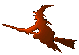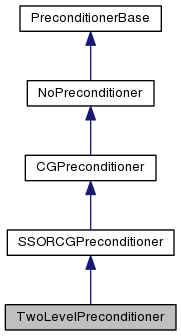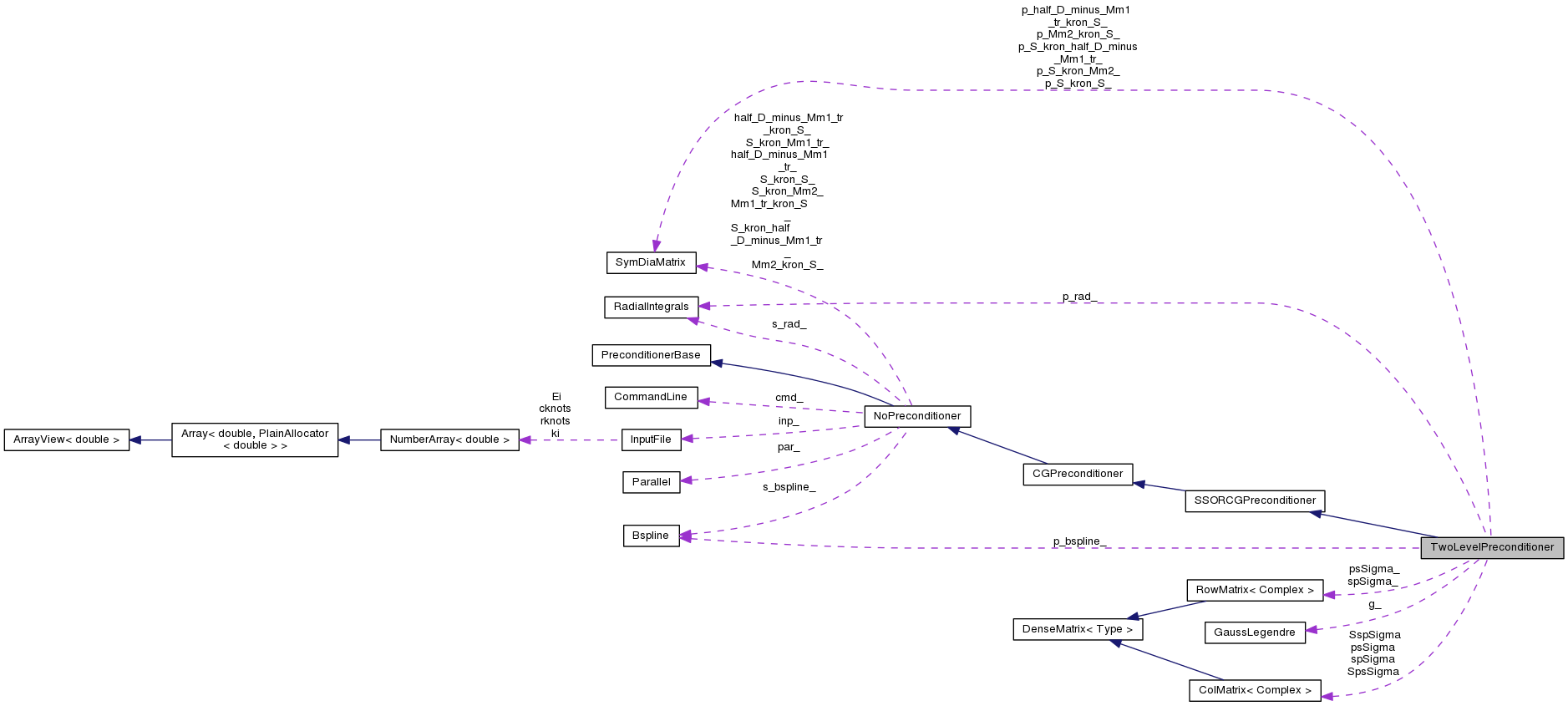Hex  1.0 Hydrogen-electron collision solver
TwoLevelPreconditioner Class Reference

Two-resolution preconditioner. More...

#include <preconditioners.h>

Inheritance diagram for TwoLevelPreconditioner:[legend]
Collaboration diagram for TwoLevelPreconditioner:[legend]

## Public Member Functions

TwoLevelPreconditioner (Parallel const &par, InputFile const &inp, std::vector< std::pair< int, int >> const &ll, Bspline const &s_bspline, CommandLine const &cmd)

RadialIntegrals const & rad () const
Get radial integrals. More...

void precondition (const cArrayView r, cArrayView z) const
Precondition the equation. More...

void setup ()
Initialize the preconditioner. More...

void update (double E)
Update the preconditioner for the next energy. More...

void rhs (cArrayView chi, int ienergy, int instate) const
Return the right-hand side. More...

void multiply (const cArrayView p, cArrayView q) const
Multiply by the matrix equation. More...

virtual void CG_prec (int iblock, const cArrayView r, cArrayView z) constPublic Member Functions inherited from SSORCGPreconditioner
SSORCGPreconditioner (Parallel const &par, InputFile const &inp, std::vector< std::pair< int, int >> const &ll, Bspline const &bspline, CommandLine const &cmd)Public Member Functions inherited from CGPreconditioner
CGPreconditioner (Parallel const &par, InputFile const &inp, std::vector< std::pair< int, int >> const &ll, Bspline const &bspline, CommandLine const &cmd)

virtual void CG_mmul (int iblock, const cArrayView p, cArrayView q) constPublic Member Functions inherited from NoPreconditioner
NoPreconditioner (Parallel const &par, InputFile const &inp, std::vector< std::pair< int, int >> const &ll, Bspline const &bspline, CommandLine const &cmd)

## Static Public Attributes

static const std::string name

static const std::string descriptionStatic Public Attributes inherited from SSORCGPreconditioner
static const std::string name = "SSOR"

static const std::string description = "Block inversion using SSOR-preconditioned conjugate gradients."Static Public Attributes inherited from CGPreconditioner
static const std::string name = "cg"

static const std::string description = "Block inversion using plain conjugate gradients."Static Public Attributes inherited from NoPreconditioner
static const std::string name = "none"

static const std::string description = "Preconditioning by the identity matrix."

## Protected Member Functions

void computeSigma_ ()

Complex computeSigma_iknot_ (int qord, int is, int iknots, int ip, int iknotp) const

## Protected Attributes

RowMatrix< ComplexspSigma_

RowMatrix< ComplexpsSigma_

ColMatrix< ComplexspSigma
DEBUG. More...

ColMatrix< ComplexSspSigma

ColMatrix< ComplexpsSigma

ColMatrix< ComplexSpsSigma

Bspline p_bspline_

RadialIntegrals p_rad_

std::vector< CsrMatrixp_csr_

std::vector< CsrMatrix::LUftp_lu_

SymDiaMatrix p_half_D_minus_Mm1_tr_kron_S_

SymDiaMatrix p_S_kron_half_D_minus_Mm1_tr_

SymDiaMatrix p_Mm2_kron_S_

SymDiaMatrix p_S_kron_Mm2_

SymDiaMatrix p_S_kron_S_

GaussLegendre g_Protected Attributes inherited from SSORCGPreconditioner
std::vector< SymDiaMatrixSSOR_Protected Attributes inherited from NoPreconditioner
CommandLine const & cmd_

Parallel const & par_

InputFile const & inp_

std::vector< std::pair< int,
int > > const &
l1_l2_

std::vector< SymDiaMatrixdia_blocks_

Bspline s_bspline_

RadialIntegrals s_rad_

SymDiaMatrix S_kron_S_

SymDiaMatrix S_kron_Mm1_tr_

SymDiaMatrix S_kron_Mm2_

SymDiaMatrix Mm1_tr_kron_S_

SymDiaMatrix Mm2_kron_S_

SymDiaMatrix half_D_minus_Mm1_tr_

SymDiaMatrix half_D_minus_Mm1_tr_kron_S_

SymDiaMatrix S_kron_half_D_minus_Mm1_tr_

## Detailed Description

This preconditioner class is inspired by the method of multi-resolution in the finite difference computations. A high-order differential operator that gives rise to the multi-diagonal matrix is initially solved in low order. The result is then used as a preconditioner for the full, high-order method.

In the case of B-spline expansion, that is being used in Hex, the bandwidth of the resulting matrix is given by the order of the B-splines. Analogously to the just metioned method, we compute the solution in a sharper base (lower order), which is computationally less demanding. The algorithm works as follows:

• In every step we are solving the equation $$\mathbf{M}^{(s)}\mathbf{z}^{(s)} = \mathbf{r}^{(s)}$$.
• The matrix $$\mathbf{M}^{(s)}$$ is the diagonal block $$E\mathbf{S}^{(s)}\otimes\mathbf{S}^{(s)} - \mathbf{H}^{(s)}$$. The symbol "s" stands for solution B-spline basis.
• But we want just to compute the simplified system $$\mathbf{M}^{(p)}\mathbf{z}^{(p)} = \mathbf{r}^{(p)}$$. The symbol "p" stands for preconditioner B-spline basis.
• The matrices contained in $$\mathbf{M}^{(p)}$$ are computed in a low-order B-spline basis; these are the matrices $$\mathbf{S}^{(p)}$$, $$\mathbf{D}^{(p)}$$ etc., whereas fhe original full-order matrices are $$\mathbf{S}^{(s)}$$, $$\mathbf{D}^{(s)}$$ etc.
• Before and after the solution of the simplified system we need to map the solutions between the bases. This is done by the equations

$\mathbf{S}^{(p)}\otimes\mathbf{S}^{(p)} \mathbf{r}^{(p)} = \mathbb{\Sigma}^{(p,q)} \otimes \mathbb{\Sigma}^{(p,q)} \mathbf{r}^{(q)}$

• Together we have the sequence

$\mathbf{S}^{(p)}\otimes\mathbf{S}^{(p)} \mathbf{r}^{(p)} = \mathbb{\Sigma}^{(p,s)} \otimes \mathbb{\Sigma}^{(p,s)} \mathbf{r}^{(s)}$

$\mathbf{M}^{(p)}\mathbf{z}^{(p)} = \mathbf{r}^{(p)}$

$\mathbf{S}^{(s)}\otimes\mathbf{S}^{(s)} \mathbf{z}^{(s)} = \mathbb{\Sigma}^{(s,p)} \otimes \mathbb{\Sigma}^{(s,p)} \mathbf{z}^{(p)}$

instead of the single equation

$\mathbf{M}^{(s)}\mathbf{z}^{(s)} = \mathbf{r}^{(s)} \ .$

The transition overlap matrix $$\mathbb{\Sigma}^{(p,q)}$$ is defined in components as

$\Sigma_{ij}^{(p,q)} = \int B_i^{(p)}(r) B_j^{(q)}(r) \mathrm{d}r \ .$

The solution of transition equations can be recast into a matrix form of Kronecker product,

$\mathbf{S}^{(p)} \mathbf{R}^{(p)} \mathbf{S}^{(p)T} = \mathbb{\Sigma}^{(p,q)}\mathrm{Matrix}(\mathbf{r}^{(q)})\mathbb{\Sigma}^{(p,q)^T} \qquad\Rightarrow\qquad \mathbf{R}^{(p)} = \mathbf{S}^{(p)^{-1}} \mathbb{\Sigma}^{(p,q)} \mathrm{Matrix}(\mathbf{r}^{(q)}) \mathbb{\Sigma}^{(p,q)^T} \mathbf{S}^{(p)^{-T}}$

where the word "Matrix" indicates that we want to split long vector into a square matrix. The resulting square matrix $$\mathbf{R}$$ will then have the same structure.

## Constructor & Destructor Documentation

 TwoLevelPreconditioner::TwoLevelPreconditioner ( Parallel const & par, InputFile const & inp, std::vector< std::pair< int, int >> const & ll, Bspline const & s_bspline, CommandLine const & cmd )
inline

## Member Function Documentation

 virtual void TwoLevelPreconditioner::CG_prec ( int iblock, const cArrayView r, cArrayView z ) const
virtual

Reimplemented from SSORCGPreconditioner.

 void TwoLevelPreconditioner::computeSigma_ ( )
protected
 Complex TwoLevelPreconditioner::computeSigma_iknot_ ( int qord, int is, int iknots, int ip, int iknotp ) const
protected
 void TwoLevelPreconditioner::multiply ( const cArrayView p, cArrayView q ) const
virtual

This function implements matrix multiplication by the matrix of the set of equations that is to be solved.

Reimplemented from SSORCGPreconditioner.

 void TwoLevelPreconditioner::precondition ( const cArrayView r, cArrayView z ) const
inlinevirtual

This function preconditions the equation, solving the preconditioner equation

$\mathbf{M}\mathbf{z} = \mathbf{r} \ .$

It may use the MPI environment.

Reimplemented from SSORCGPreconditioner.

 RadialIntegrals const& TwoLevelPreconditioner::rad ( ) const
inlinevirtual

Reimplemented from SSORCGPreconditioner.

 void TwoLevelPreconditioner::rhs ( cArrayView chi, int ienergy, int instate ) const
virtual

Reimplemented from SSORCGPreconditioner.

 void TwoLevelPreconditioner::setup ( )
virtual

This function contains all computation intensive preparations for the preconditioner, e.g. computation of radial integrals. It may use only SMP environment.

Reimplemented from SSORCGPreconditioner.

 void TwoLevelPreconditioner::update ( double E )
virtual

This function updates the preconditioner for another right hand side. It may use the MPI environment.

Reimplemented from SSORCGPreconditioner.

## Field Documentation

 const std::string TwoLevelPreconditioner::description
static
 GaussLegendre TwoLevelPreconditioner::g_
protected
 const std::string TwoLevelPreconditioner::name
static
 Bspline TwoLevelPreconditioner::p_bspline_
protected
 std::vector TwoLevelPreconditioner::p_csr_
protected
 SymDiaMatrix TwoLevelPreconditioner::p_half_D_minus_Mm1_tr_kron_S_
protected
 std::vector TwoLevelPreconditioner::p_lu_
protected
 SymDiaMatrix TwoLevelPreconditioner::p_Mm2_kron_S_
protected
 RadialIntegrals TwoLevelPreconditioner::p_rad_
protected
 SymDiaMatrix TwoLevelPreconditioner::p_S_kron_half_D_minus_Mm1_tr_
protected
 SymDiaMatrix TwoLevelPreconditioner::p_S_kron_Mm2_
protected
 SymDiaMatrix TwoLevelPreconditioner::p_S_kron_S_
protected
 ColMatrix TwoLevelPreconditioner::psSigma
protected
 RowMatrix TwoLevelPreconditioner::psSigma_
protected
 ColMatrix TwoLevelPreconditioner::spSigma
protected
 RowMatrix TwoLevelPreconditioner::spSigma_
protected
 ColMatrix TwoLevelPreconditioner::SpsSigma
protected
 ColMatrix TwoLevelPreconditioner::SspSigma
protected

The documentation for this class was generated from the following file: